or# RD Sharma Class 10 and Chapter Circles Maths Solutions

## Maths Class 10 and Chapter Circles RD Sharma SolutionsFilter
Filters :
CLASSES
•
•
•
•
•
•
•
CHAPTERS
•
• 6 More

### RD SHARMA Class 10 | CIRCLES

If radii of the two concentric circles are 15cm and 17cm, then the length of each chord of one circle which is tangent to other is:   8cm       (b)   16cm   (c)  30cm    (d) 17cm### RD SHARMA Class 10 | CIRCLES

If from a point `P ,` tangents `P Qa n dP R` are drawn to the ellipse `(x^2)/2+y^2=1` so that the equation of `Q R` is `x+3y=1,` then find the coordinates of `Pdot`### RD SHARMA Class 10 | CIRCLES

In Figure, if tangents `P Aa n dP B` are drawn to a circle such that `/_A P B=30^0` and chord `A C` is drawn parallel to the tangent `P B ,` then `/_A B C=` `60^0` (b) `90^0` (c) `30^0` (d) None of these### RD SHARMA Class 10 | CIRCLES

In two concentric circles, a chord of length 24cm of larger circle becomes a tangent to the smaller circle whose radius is 5cm. Find the radius of the larger circle.In this chapter, we will learn about Circles and the details on Tangents of Circle. Several theorems and solved problems based on the properties of the circle are provided in this chapter. Doubtnut has made it much easier for the students to understand the subject with the help of the video tutorials. The theorems and solved problems are presented in a simple way that students can understand easily. Since following a theorem is crucial for scoring good marks in examination, so the video solutions are prepared in such a way so that students won’t find any difficulties while looking at the examples and will be able to solve all problems with ease.

It is observed that students following the NCERT textbooks find it quite perplexing in getting an in-depth understanding of various concepts. Math is such a subject that demands rigorous practise for achieving success. RD Sharma books help students to a certain extent in getting some good idea on how to clear their queries. But this does not lead to getting all clear ideas where they fail to attempt the tricky questions that are set in the CBSE board exams. This leads the students to get low marks in the subject, which leads to finding themselves low confident as well.

Owing to the various complications or problems faced by the students, a team of experts of Doubtnut has introduced online study materials. This makes it much easier for them to find all the essential and useful idea on how to solve even the trickiest questions without fail. Therefore, it can be the right choice for the students to opt for it if they genuinely wish to gain good marks in the examination. So, it becomes possible to practice in the best way with the help of various examples and exercises. It is possible to get the best solution with the support of the RD Sharma Solutions class 10 maths. The concepts explained in the video tutorials are helpful in preparation of engineering entrance examination like JEE Main and Advanced.

## Topics and subtopics of RD Sharma class 10 Chapter 10

Introduction: Since you have studied already about Circles, so we will mainly focus on Tangents and practice on the same with several examples and problems. Here in this chapter, various concepts like tangents, tangents to a circle, the number of tangents from a point on a circle, etc. are introduced. For the convenience of the students, several solved examples are provided here to clear these concepts and to solve the problems quickly.

Some important point to remember:

1) A circle can have infinite tangents (Tangent is a line that intersects the circle at one point. There is infinite number of points on a circle).

2) Secant is a line that intersects a circle in two points.

3) The Point of contact is a common point of a tangent to a circle and the circle.

Theorems are given below:

### Theorem 1

The tangent at any point of a circle is perpendicular to the radius through the point of contact.

A circle is given with its center point “C”. “AB” is a tangent at the point of contact“X”. Prove that CX is perpendicular to AB.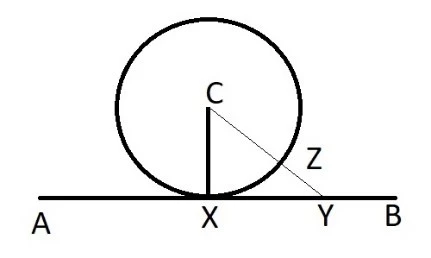Proof:

Let Y be a point on AB.

Connect CY. Suppose it touches the circle at Z

Hence,

CY > CZ

CY > CX (since, CX = CZ = radius)

Therefore, it will be same with any other points on the circle.

Let us try an exercise:

“AB” is a tangent of a circle at a point “X”. AB meets a line CY at point Y. The radius CX = 6 cm. The line CY = 14 cm. Find out the length of XY.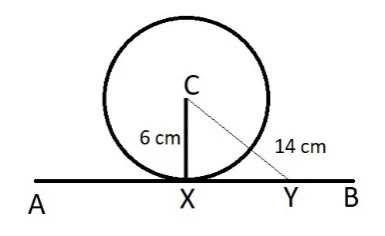Solution:

Given, CX = radius = 6 cm

CY = 14 cm.

Since, XY is a tangent,

Hence, CX is perpendicular to XY(since tangent at any point of the circle is perpendicular to the radius through point of contact)

So,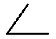CXY = 90o

Hence, Δ CXY is a right-angle triangle.

In right angle triangle, using Pythagoras theorem

(Hypotenuse)2 = (Height)2 + (Base)2

• (CY)2   = (CX)2 + (XY)2
• (14)2     = (6)2  + (XY)2
• 196       = 36    + (XY)2
• 196 – 36 = (XY)2
• (XY)2        =  160
• XY          =  √160 = 12.6 cm

### Theorem 2

The lengths of tangents drawn from an external point to a circle are equal.

A circle is drawn with its centre point C and two tangents are drawn from a point P outside the circle connecting at A and B.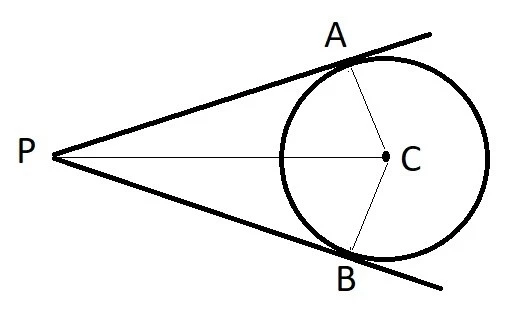Prove PA = PB

Solution: Let us connect AC, PC, and BC

As PA is tangent,

PA    |   CA  (Tangent at any point of the circle is

The point of contact

So,PAC = 90o

Hence, Δ PCA is a right-angle triangle.

Similarly,

PB is a tangent.

PB    _|_   CB  (Tangent at any point of the circle is

The point of contact

So,PBC = 90o

Hence, Δ PCB is a right-angle triangle.

In Δ PCA and Δ PCB

PC is a common line.

Since PA and PB are tangents,PAC andPBC are right angles

Therefore,

In Δ PCA and Δ PCBPAC =PBC (both are 90o)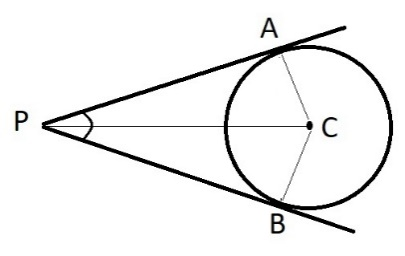PC  = PC (common line between the triangles)

CA = CB (radius of the circle)

Δ PCA Δ PCB

PA = PB

Therefore, tangents drawn from an external point to a circle are equal in lengths.

Let’s do an exercise to understand the above theorem:

• If PA and PB are tangents from a point P to the circle

with centre Cand the angle between PA and PB is

600, then what will bePCA =?Solution: Given       BPA = 600

Connect P, A, B, and C first.

Since PA is a tangent to the circle.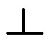CA      PA(  Tangent at any point of circle is perpendicular to the radius through

the point of contact

so,          CAP = 90o

In Δ PCA and Δ PCB600PAC =PBC  (both are 90o)

PC  = PC  (common line between the triangles)

CA  = CB  (radius of the circle)

Δ PCA Δ PCB

So,CPA =CPB

= ½BPA

= ½ x 60o

= 30o

In triangle Δ PCA,PCA +PAC +CPA = 180o

•PCA+  90o   + 30o     = 180o
•PCA              = 180o- 90o- 30o= 60o Hence proved.

Some exercises are given below:

Exercise: In two concentric circles, the chord of the larger circle which touches the smaller circle is bisected at the point of contact. Prove it.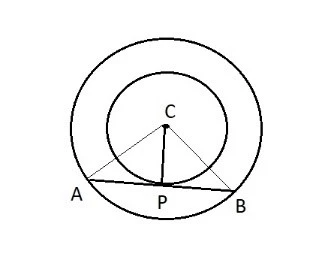Solution: Let us take two concentric circles.

Where AB is the chord of the larger circle which touches the

smaller circle at P. Connect the centre C and P. Now to

Prove chord AB is bisected at the point P i.e. AP = PB

Since AB is tangent to the smaller circle

So, CP  _|_  AB  (Tangent at any point of circle is perpendicular to the radius through

the point of contact)

Now, connect CA and CB.

In Δ APC and Δ BPC,CPA =CPB  (both are 90o)

PC  = PC  (common line between the triangles)

CA  = CB  (radius of the circle)

Δ APC ΔBPC

Therefore,     AP  = PB Hence proved.

Exercise: Two concentric circles are of radii 7 cm and 5 cm. Find the length of the chord of the larger circle which touches the smaller circle. Solution:

Solution: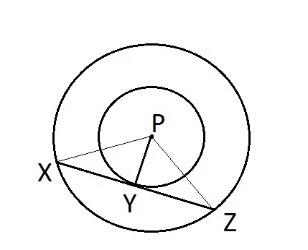Let the centre of the two concentric circles be P and chord XY

of the larger circle touches the smaller perplexing at Y. Since XZ is

tangent to the smaller circle, hence PY XZ.

Given,

PY = 3 cm

PX = 5 cm

Applying Pythagoras theorem in PXY,

PY2 + XY2 = PX2

9 + XY2 = 25

XY2 = 25 - 9

XY2 = 16 cm = 4 cm

Since,

XY = YZ   (Perpendicular from centre of circle bisects the chord)

XZ = 2XY = 2 × 4 cm = 8 cm

Hence, length of chord of larger circle is 8 cm.

Exercise: The tangents drawn at the ends of a diameter of a circle are parallel. Prove this.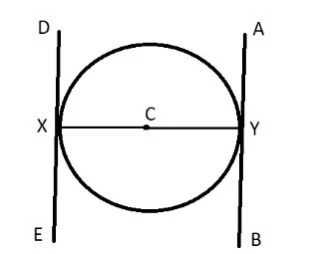Solution:  Let us take a circle with centre C. XY is its diameter. AB and DE are two tangents of the circle connect at the endpoints X and Y. Now we have to prove AB is parallel to DE.

As we learned already that the radius is perpendicular to the tangent at the point of contact. Hence CYA = 90o and CXD = 90o

Again,CYB = CXE= 90o

Since the opposite angles are equal, hence the tangents must be parallel to each other.

Since alternate interior angles are equal, hence lines PQ and RS must be parallel.

## Importance of Doubtnut for the students

The students obtain all the critical concepts and comprehensive understanding of how to solve tricky questions. R D Sharma Solutions for Class 10 Maths Chapter 10 Circles make things much easier for them to get a better idea on how questions are set in the examination. Some of the advantages that students can enjoy by opting for the video tutorials are as follows:

• Easy to understand: Students are able to get a fundamental understanding of the subject as it is prepared using simple and lucid language. With the best in-depth video solutions, students can attempt different questions without any problem at all.
• No need to pay any money: All the online study materials provided to the students are free to access. It can also be downloaded easily in the pdf format which makes it flexible for the students to learn according to their own time. So, it can help a lot to find all the vital information on how to deal with different questions without wasting a lot of time at all.
• Provides the best support: If students find it challenging to understand how to solve a particular question, then Doubtnut can provide them with the best support. Here, students do not have to find themselves confused at all as the experts are there to get them cleared without any difficulty in understanding it. So, Doubtnut can genuinely help students to develop a liking for the subject by providing them with all the best idea on how to work on it without any problem.